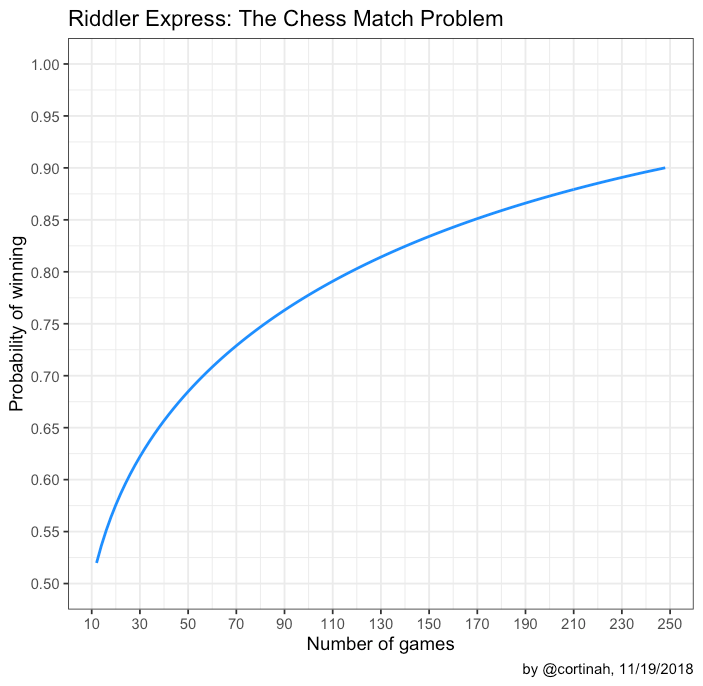Want to share your content on R-bloggers? click here if you have a blog, or here if you don't.

### The Problem

This week’s Riddler Express was more involved than I anticipated.

The World Chess Championship is underway. We need to find the odds that the better player wins a 12-game match, given:

One of the players is better than his opponent to the degree that he wins 20% of all games, loses 15%, and 65% of games are drawn. Wins are worth 1 point, draws 0.5 for each player, and losses 0 points. In a 12-game match, the first player to earn 6.5 points wins.

How many games would a match have to be in order to give the better player a 75% chance of winning the match outright? A 90% chance? A 99% chance?

### Approach

The approach is to count all of the ways the player can win the match, the probability of each of the win scenarios, and sum the product of these two across all possible wins. It helps to illustrate this with a table for the 12-game match case:Where W is # of games won, D is # of draws, and L # of losses. For example, the player can win the match with 10 wins, 1 draw, and 1 loss, or 4 wins, 6 draws and 2 losses.

To obtain the probability of each of these win scenarios, we count the number of ways it can occur times its probability. Specifically:

$P= \binom{12}{win} \binom{12-win}{draw} 0.20^{win} 0.15^{lose}0.65^{draw}$

I initially did the calculation on a spreadsheet and obtained 0.5198 as the answer, but using a spreadsheet doesn’t work for the second part of the problem.## Finding the number of games in a match such that P > alpha

With a 12-game match the stronger player can only be expected to win around the match 52% of the time, because his chess ability advantage is relatively small. This is unsatisfying because the stronger chess player will still lose the match nearly half of the time due to randomness. (PS: that’s also my excuse when my king falls.)

Accordingly, how many games does it take for the better player to win 75%, 90%, and 99% of the time. I coded a function in R to calculate this, which is available here.

The function performs the same calculation as the spreadsheet, for any even number of games.

We test that we obtain the same answer as the spreadsheet for 12 games:

chess(12)

 0.5198

With a little trial and error, we find the number of games for 75%, 90%, and 99% probability the better player wins:

chess(82)

 0.7503

chess(248)

 0.9001

chess(772)

 0.99

Which we can also illustrate with a graph showing the large number of games necessary to obtain a high degree of confidence that the results of a match were not a fluke!Now let’s get back to Carlsen-Caruana.